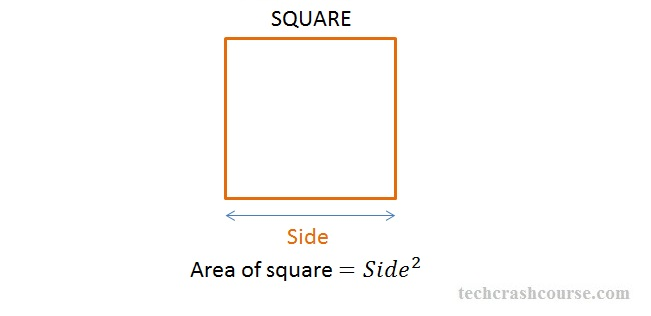# C Program Area Of Square | C Programs

C Program for calculating the area of a square – In this particular article, we will brief in on all the methods used to calculate the area of a square.

The ways to calculate the area of a square are as follows:

• Using Standard Method
• Using Function
• Using Pointers
• Using Macros

Check out the blog for the solutions. We have added the compiler to help you out with the C programming code. Also, check out the sample outputs and suitable examples pertaining to the respective codes.

As we all know, a square is a closely bound 2-dimensional figure made up of four sides. A square has a bunch of impressive traits. All the sides of a square are equal in nature.

Even all the angles of a square are also equal in nature. Since the sum of all angles of any quadrilateral is equal to 360 degrees. All the angles of a square are equal to 90 degrees.

A square would look like this:Source

As you can see in the image uploaded above, the area of a square can be calculated by squaring the length of a side.

The distinct ways to calculate the area of a square are as follows:

## Using Standard Method

1) For calculating the area of the square, we need a length of the side.

2) The side value will store into the variable “side”.

3) The value of side will substituted int the formula then we will get area value,that value will assign to the variable “area”.

output:

### Using Function

1)float area(float s) calculates the area of square. This function is called as “called function”.

2)We are calling the function using the code area(s).

3)The called function will calculate the area and returns the value,that value will assigned to the variable “a”.

output:

#### Using Pointers

1)We are calling the function by passing the references as arguments in the code area(&s,&a).

2)The called function retrieve the values from the given addresses and calculate  the area,and that value will store into the pointer variable “area”.

output:

##### Using Macros

1)area(s) is symbolic name to the expression (s*s).

2)At the code area(s)replaced with that expression given at #define.

output:
x

## C Program To Print Number Of Days In A Month | Java Tutoring

C program to input the month number and print the number of days in that ...